## 5.49. balance_tree

Origin
Constraint

$\mathrm{𝚋𝚊𝚕𝚊𝚗𝚌𝚎}_\mathrm{𝚝𝚛𝚎𝚎}\left(\mathrm{𝙱𝙰𝙻𝙰𝙽𝙲𝙴},\mathrm{𝙽𝙾𝙳𝙴𝚂}\right)$

Arguments
 $\mathrm{𝙱𝙰𝙻𝙰𝙽𝙲𝙴}$ $\mathrm{𝚍𝚟𝚊𝚛}$ $\mathrm{𝙽𝙾𝙳𝙴𝚂}$ $\mathrm{𝚌𝚘𝚕𝚕𝚎𝚌𝚝𝚒𝚘𝚗}\left(\mathrm{𝚒𝚗𝚍𝚎𝚡}-\mathrm{𝚒𝚗𝚝},\mathrm{𝚜𝚞𝚌𝚌}-\mathrm{𝚍𝚟𝚊𝚛}\right)$
Restrictions
 $\mathrm{𝙱𝙰𝙻𝙰𝙽𝙲𝙴}\ge 0$ $\mathrm{𝙱𝙰𝙻𝙰𝙽𝙲𝙴}\le \mathrm{𝚖𝚊𝚡}\left(0,|\mathrm{𝙽𝙾𝙳𝙴𝚂}|-2\right)$ $\mathrm{𝚛𝚎𝚚𝚞𝚒𝚛𝚎𝚍}$$\left(\mathrm{𝙽𝙾𝙳𝙴𝚂},\left[\mathrm{𝚒𝚗𝚍𝚎𝚡},\mathrm{𝚜𝚞𝚌𝚌}\right]\right)$ $\mathrm{𝙽𝙾𝙳𝙴𝚂}.\mathrm{𝚒𝚗𝚍𝚎𝚡}\ge 1$ $\mathrm{𝙽𝙾𝙳𝙴𝚂}.\mathrm{𝚒𝚗𝚍𝚎𝚡}\le |\mathrm{𝙽𝙾𝙳𝙴𝚂}|$ $\mathrm{𝚍𝚒𝚜𝚝𝚒𝚗𝚌𝚝}$$\left(\mathrm{𝙽𝙾𝙳𝙴𝚂},\mathrm{𝚒𝚗𝚍𝚎𝚡}\right)$ $\mathrm{𝙽𝙾𝙳𝙴𝚂}.\mathrm{𝚜𝚞𝚌𝚌}\ge 1$ $\mathrm{𝙽𝙾𝙳𝙴𝚂}.\mathrm{𝚜𝚞𝚌𝚌}\le |\mathrm{𝙽𝙾𝙳𝙴𝚂}|$
Purpose

Consider a digraph $G$ described by the $\mathrm{𝙽𝙾𝙳𝙴𝚂}$ collection. Partition $G$ into a set of vertex disjoint trees in such a way that each vertex of $G$ belongs to a single tree. $\mathrm{𝙱𝙰𝙻𝙰𝙽𝙲𝙴}$ is equal to the difference between the number of vertices of the largest tree and the number of vertices of the smallest tree.

Example
 $\left(\begin{array}{c}4,〈\begin{array}{cc}\mathrm{𝚒𝚗𝚍𝚎𝚡}-1\hfill & \mathrm{𝚜𝚞𝚌𝚌}-1,\hfill \\ \mathrm{𝚒𝚗𝚍𝚎𝚡}-2\hfill & \mathrm{𝚜𝚞𝚌𝚌}-5,\hfill \\ \mathrm{𝚒𝚗𝚍𝚎𝚡}-3\hfill & \mathrm{𝚜𝚞𝚌𝚌}-5,\hfill \\ \mathrm{𝚒𝚗𝚍𝚎𝚡}-4\hfill & \mathrm{𝚜𝚞𝚌𝚌}-7,\hfill \\ \mathrm{𝚒𝚗𝚍𝚎𝚡}-5\hfill & \mathrm{𝚜𝚞𝚌𝚌}-1,\hfill \\ \mathrm{𝚒𝚗𝚍𝚎𝚡}-6\hfill & \mathrm{𝚜𝚞𝚌𝚌}-1,\hfill \\ \mathrm{𝚒𝚗𝚍𝚎𝚡}-7\hfill & \mathrm{𝚜𝚞𝚌𝚌}-7,\hfill \\ \mathrm{𝚒𝚗𝚍𝚎𝚡}-8\hfill & \mathrm{𝚜𝚞𝚌𝚌}-5\hfill \end{array}〉\hfill \end{array}\right)$ $\left(\begin{array}{c}2,〈\begin{array}{cc}\mathrm{𝚒𝚗𝚍𝚎𝚡}-1\hfill & \mathrm{𝚜𝚞𝚌𝚌}-1,\hfill \\ \mathrm{𝚒𝚗𝚍𝚎𝚡}-2\hfill & \mathrm{𝚜𝚞𝚌𝚌}-1,\hfill \\ \mathrm{𝚒𝚗𝚍𝚎𝚡}-3\hfill & \mathrm{𝚜𝚞𝚌𝚌}-1,\hfill \\ \mathrm{𝚒𝚗𝚍𝚎𝚡}-4\hfill & \mathrm{𝚜𝚞𝚌𝚌}-2,\hfill \\ \mathrm{𝚒𝚗𝚍𝚎𝚡}-5\hfill & \mathrm{𝚜𝚞𝚌𝚌}-6,\hfill \\ \mathrm{𝚒𝚗𝚍𝚎𝚡}-6\hfill & \mathrm{𝚜𝚞𝚌𝚌}-6\hfill \end{array}〉\hfill \end{array}\right)$

In the first example we have two trees involving respectively the set of vertices $\left\{1,2,3,5,6,8\right\}$ and the set $\left\{4,7\right\}$. They are depicted by Figure 5.49.1. Since $\mathrm{𝙱𝙰𝙻𝙰𝙽𝙲𝙴}=6-2=4$ is the difference between the number of vertices of the largest tree (i.e., 6) and the number of vertices of the smallest tree (i.e., 2) the corresponding $\mathrm{𝚋𝚊𝚕𝚊𝚗𝚌𝚎}_\mathrm{𝚝𝚛𝚎𝚎}$ constraint holds.

##### Figure 5.49.1. The two trees associated with the first example of the Example slot, respectively containing 6 and 2 vertices, therefore $\mathrm{𝙱𝙰𝙻𝙰𝙽𝙲𝙴}=6-2=4$; each vertex contains the information $\mathrm{𝚒𝚗𝚍𝚎𝚡}|\mathrm{𝚜𝚞𝚌𝚌}$ where $\mathrm{𝚜𝚞𝚌𝚌}$ is the index of its father in the tree (by convention the father of the root is the root itself).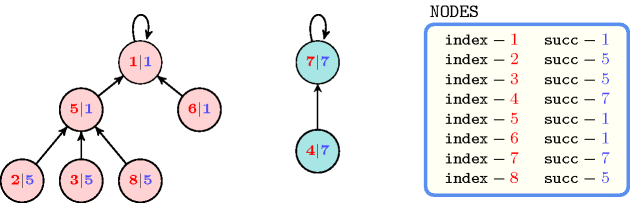All solutions

Figure 5.49.2 gives all solutions to the following non ground instance of the $\mathrm{𝚋𝚊𝚕𝚊𝚗𝚌𝚎}_\mathrm{𝚝𝚛𝚎𝚎}$ constraint: $\mathrm{𝙱𝙰𝙻𝙰𝙽𝙲𝙴}=0$, ${S}_{1}\in \left[1,2\right]$, ${S}_{2}\in \left[1,2\right]$, ${S}_{3}\in \left[4,5\right]$, ${S}_{4}\in \left[2,4\right]$, ${S}_{5}\in \left[4,5\right]$, ${S}_{6}\in \left[5,6\right]$, $\mathrm{𝚋𝚊𝚕𝚊𝚗𝚌𝚎}_\mathrm{𝚝𝚛𝚎𝚎}$$\left(\mathrm{𝙱𝙰𝙻𝙰𝙽𝙲𝙴},〈1{S}_{1},2{S}_{2},3{S}_{3},4{S}_{4},5{S}_{5},6{S}_{6}〉\right)$.

##### Figure 5.49.2. All solutions corresponding to the non ground example of the $\mathrm{𝚋𝚊𝚕𝚊𝚗𝚌𝚎}_\mathrm{𝚝𝚛𝚎𝚎}$ constraint of the All solutions slot; the $\mathrm{𝚒𝚗𝚍𝚎𝚡}$ attribute is displayed as indices of the $\mathrm{𝚜𝚞𝚌𝚌}$ attribute and all vertices of a same tree are coloured by the same colour.Typical
$|\mathrm{𝙽𝙾𝙳𝙴𝚂}|>2$
Symmetry

Items of $\mathrm{𝙽𝙾𝙳𝙴𝚂}$ are permutable.

Arg. properties

Functional dependency: $\mathrm{𝙱𝙰𝙻𝙰𝙽𝙲𝙴}$ determined by $\mathrm{𝙽𝙾𝙳𝙴𝚂}$.

Counting
 Length ($n$) 2 3 4 5 6 7 8 Solutions 3 16 125 1296 16807 262144 4782969

Number of solutions for $\mathrm{𝚋𝚊𝚕𝚊𝚗𝚌𝚎}_\mathrm{𝚝𝚛𝚎𝚎}$: domains $0..n$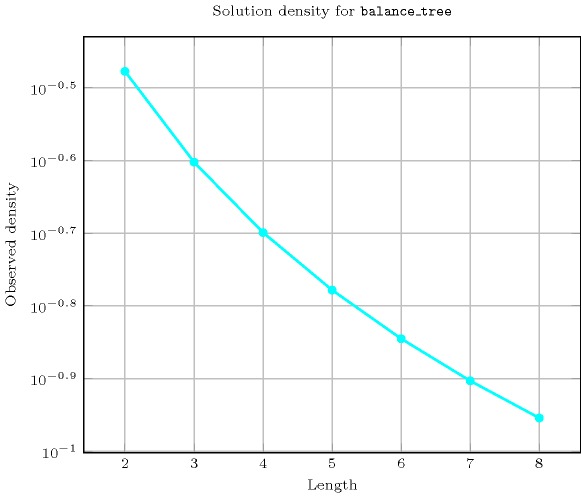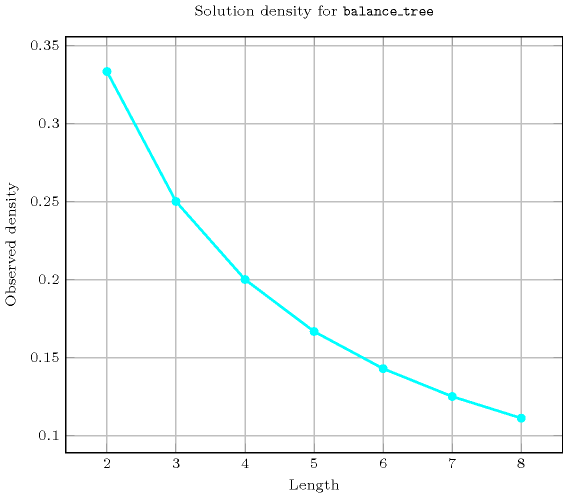Length ($n$)2345678
Total3161251296168072621444782969
 Parameter value

03107762687071176502242193
1-6122602102524249616
2--369031809765432264
3---32096041930219520
4----375013125680456
5-----54432217728
6------941192

Solution count for $\mathrm{𝚋𝚊𝚕𝚊𝚗𝚌𝚎}_\mathrm{𝚝𝚛𝚎𝚎}$: domains $0..n$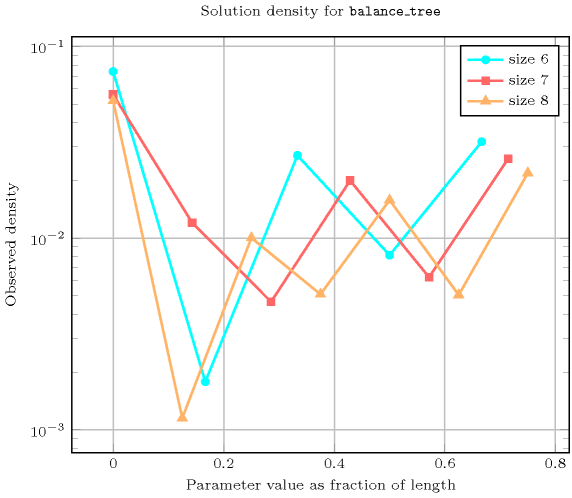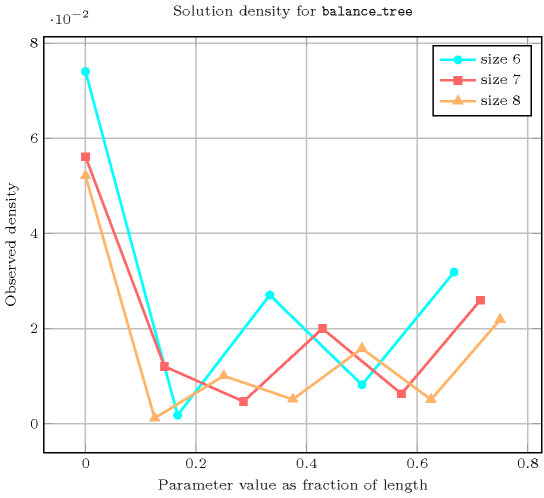related: $\mathrm{𝚋𝚊𝚕𝚊𝚗𝚌𝚎}$ (equivalence classes correspond to vertices in same tree rather than variables assigned to the same value), $\mathrm{𝚝𝚛𝚎𝚎}$ (do not care how many trees but how balanced the trees are).

Keywords
Cond. implications

$\mathrm{𝚋𝚊𝚕𝚊𝚗𝚌𝚎}_\mathrm{𝚝𝚛𝚎𝚎}\left(\mathrm{𝙱𝙰𝙻𝙰𝙽𝙲𝙴},\mathrm{𝙽𝙾𝙳𝙴𝚂}\right)$

with  $\mathrm{𝙱𝙰𝙻𝙰𝙽𝙲𝙴}>0$

and   $\mathrm{𝙱𝙰𝙻𝙰𝙽𝙲𝙴}\le |\mathrm{𝙽𝙾𝙳𝙴𝚂}|$

implies $\mathrm{𝚘𝚛𝚍𝚎𝚛𝚎𝚍}_\mathrm{𝚊𝚝𝚕𝚎𝚊𝚜𝚝}_\mathrm{𝚗𝚟𝚎𝚌𝚝𝚘𝚛}$$\left(\mathrm{𝙽𝚅𝙴𝙲}:\mathrm{𝙱𝙰𝙻𝙰𝙽𝙲𝙴},\mathrm{𝚅𝙴𝙲𝚃𝙾𝚁𝚂}:\mathrm{𝙽𝙾𝙳𝙴𝚂}\right)$.

Arc input(s)

$\mathrm{𝙽𝙾𝙳𝙴𝚂}$

Arc generator
$\mathrm{𝐶𝐿𝐼𝑄𝑈𝐸}$$↦\mathrm{𝚌𝚘𝚕𝚕𝚎𝚌𝚝𝚒𝚘𝚗}\left(\mathrm{𝚗𝚘𝚍𝚎𝚜}\mathtt{1},\mathrm{𝚗𝚘𝚍𝚎𝚜}\mathtt{2}\right)$

Arc arity
Arc constraint(s)
$\mathrm{𝚗𝚘𝚍𝚎𝚜}\mathtt{1}.\mathrm{𝚜𝚞𝚌𝚌}=\mathrm{𝚗𝚘𝚍𝚎𝚜}\mathtt{2}.\mathrm{𝚒𝚗𝚍𝚎𝚡}$
Graph property(ies)
 $•$$\mathrm{𝐌𝐀𝐗}_\mathrm{𝐍𝐒𝐂𝐂}$$\le 1$ $•$$\mathrm{𝐑𝐀𝐍𝐆𝐄}_\mathrm{𝐍𝐂𝐂}$$=\mathrm{𝙱𝙰𝙻𝙰𝙽𝙲𝙴}$

Graph model

In order to express the binary constraint that links two vertices one has to make explicit the identifier of the vertices. This is why the $\mathrm{𝚋𝚊𝚕𝚊𝚗𝚌𝚎}_\mathrm{𝚝𝚛𝚎𝚎}$ constraint considers objects that have two attributes:

• One fixed attribute $\mathrm{𝚒𝚗𝚍𝚎𝚡}$ that is the identifier of the vertex,

• One variable attribute $\mathrm{𝚜𝚞𝚌𝚌}$ that is the successor of the vertex.

We use the graph property $\mathrm{𝐌𝐀𝐗}_\mathrm{𝐍𝐒𝐂𝐂}$$\le 1$ in order to specify the fact that the size of the largest strongly connected component should not exceed one. In fact each root of a tree is a strongly connected component with a single vertex.

Parts (A) and (B) of Figure 5.49.3 respectively show the initial and final graph associated with the first example of the Example slot. Since we use the $\mathrm{𝐑𝐀𝐍𝐆𝐄}_\mathrm{𝐍𝐂𝐂}$ graph property, we show the connected components of the final graph. The constraint holds since all the vertices belong to a tree and since $\mathrm{𝙱𝙰𝙻𝙰𝙽𝙲𝙴}$ $=$ $\mathrm{𝐑𝐀𝐍𝐆𝐄}_\mathrm{𝐍𝐂𝐂}$$6-2=4$.

##### Figure 5.49.3. Initial and final graph of the $\mathrm{𝚋𝚊𝚕𝚊𝚗𝚌𝚎}_\mathrm{𝚝𝚛𝚎𝚎}$ constraint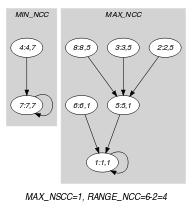(a) (b)